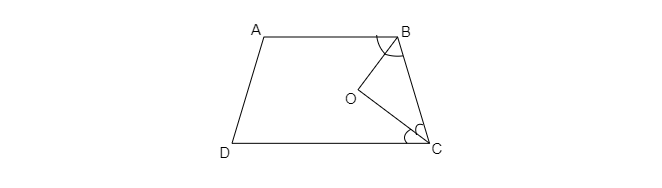"
">

# In the given figure, $A B C D$ is trapezium with $A B \| D C$. The bisectors of $\angle B$ and $\angle C$ meet at point $O$. Find $\angle B O C$."

Given: In the given figure, $A B C D$ is trapezium with $A B \| D C$. The bisectors of  $\angle B$ and $\angle C$ meet at point $O$. Find $\angle B O C$.

To do: To find $\angle BOC$.

Solution:In the given figure,

$\angle B+\angle C=180^o$

$\Rightarrow \frac{1}{2}\times \angle ABC+\frac{1}{2}\times \angle BCD=\frac{180}{2}=90^o$

$\Rightarrow \frac{\angle ABC}{2}+\frac{\angle BCD}{2}=90^o$

$\Rightarrow \angle OBC+\angle OCB=90^o$

In $\vartriangle BOC$,

$\angle OCB+\angle OBC+\angle BOC=180^o$

$\Rightarrow 90^o+\angle BOC=180^o$

$\Rightarrow \angle BOC=180^o-90^o$

$\Rightarrow \angle BOC=90^o$

Updated on: 10-Oct-2022

128 Views